# RD Sharma Solutions Class 12 Linear Programming Exercise 30.5

RD Sharma Solutions for Class 12 Maths Chapter 30 Linear Programming Exercise 30.5, is given here. Highly experienced subject experts at BYJU’S having vast knowledge of concepts have developed the solutions, which match the understanding ability of the students. To achieve a good academic score in Mathematics, students are required to practice all the questions given in this exercise. Students can easily download the RD Sharma Solutions pdf consisting of this chapter solutions, which are available in the links provided below.

## Download PDF of RD Sharma Solutions for Class 12 Maths Chapter 30 Exercise 5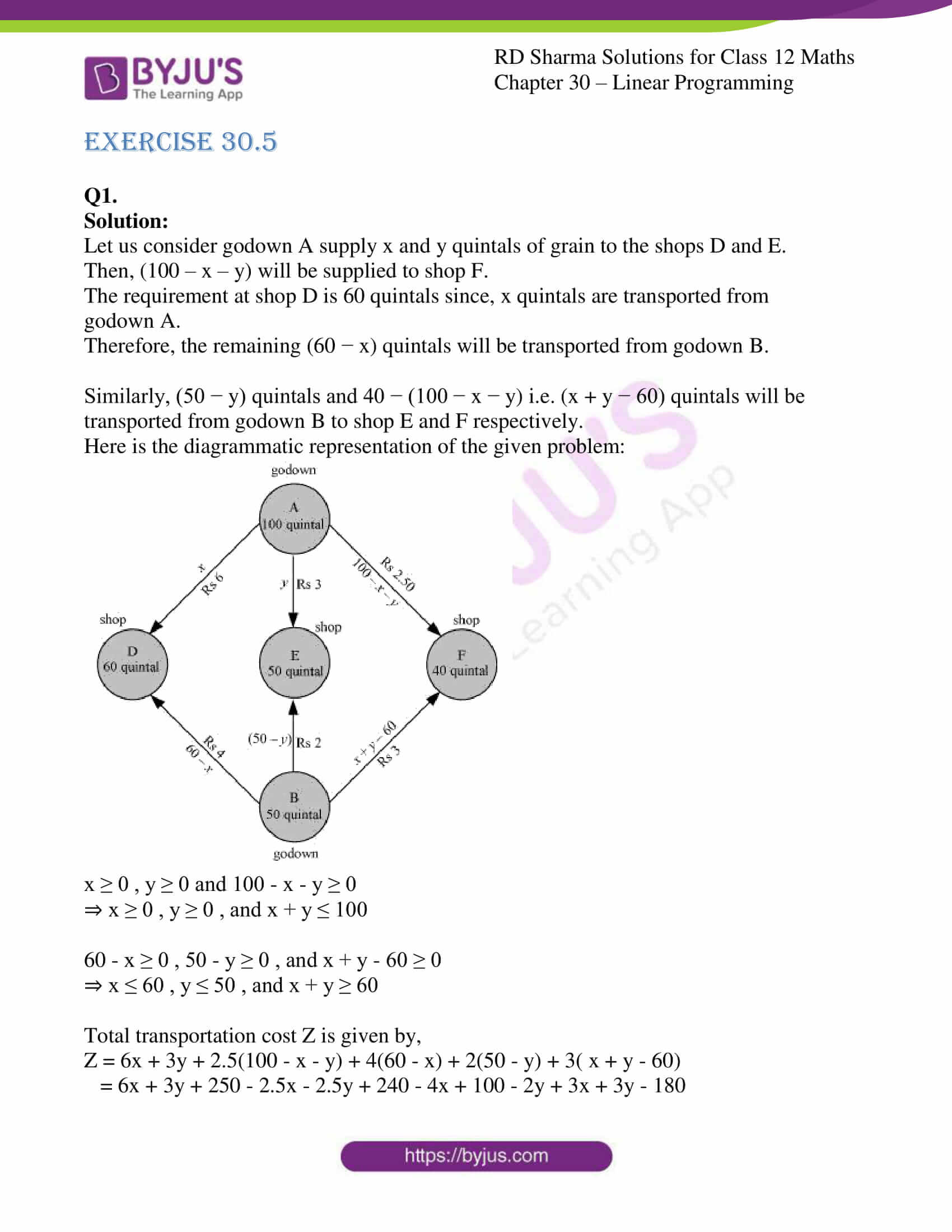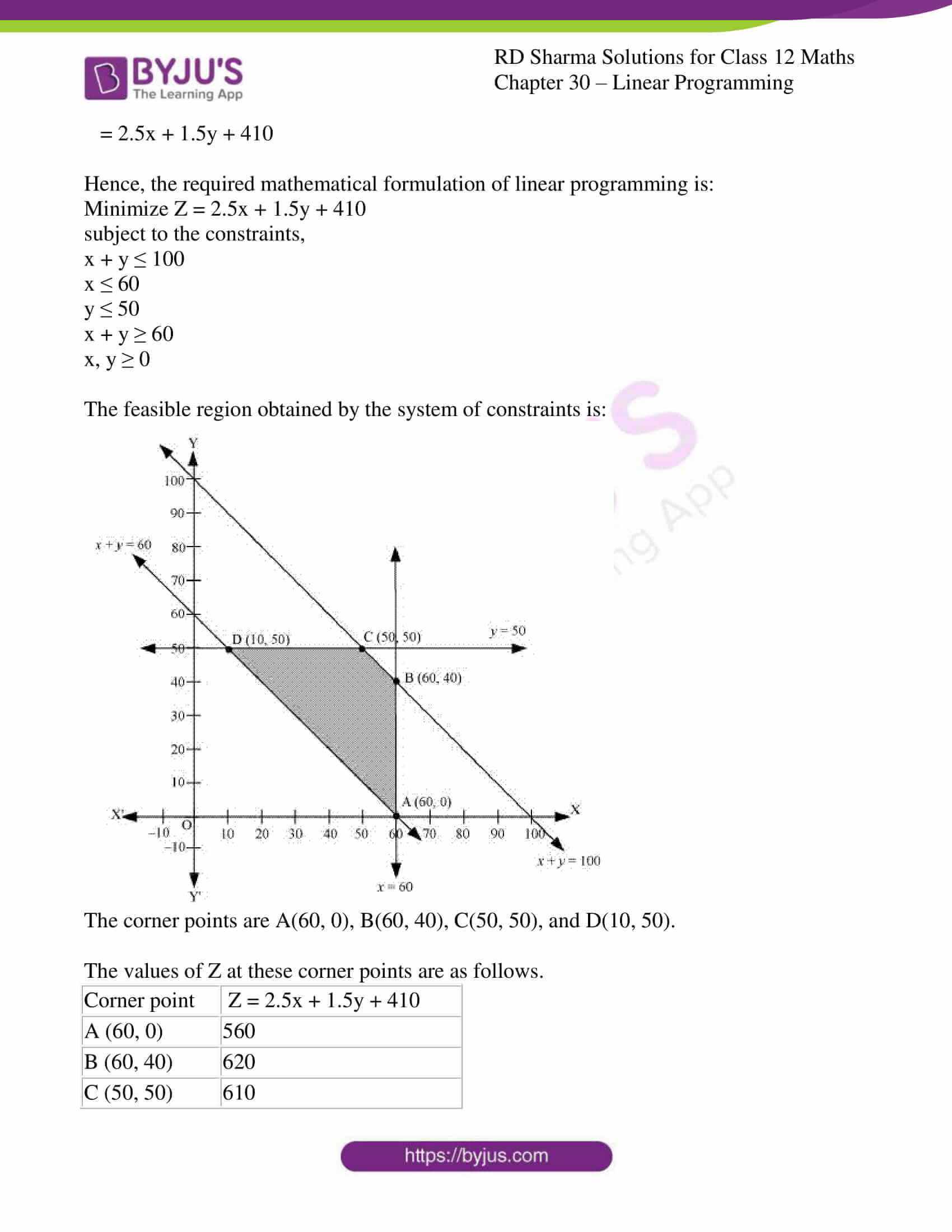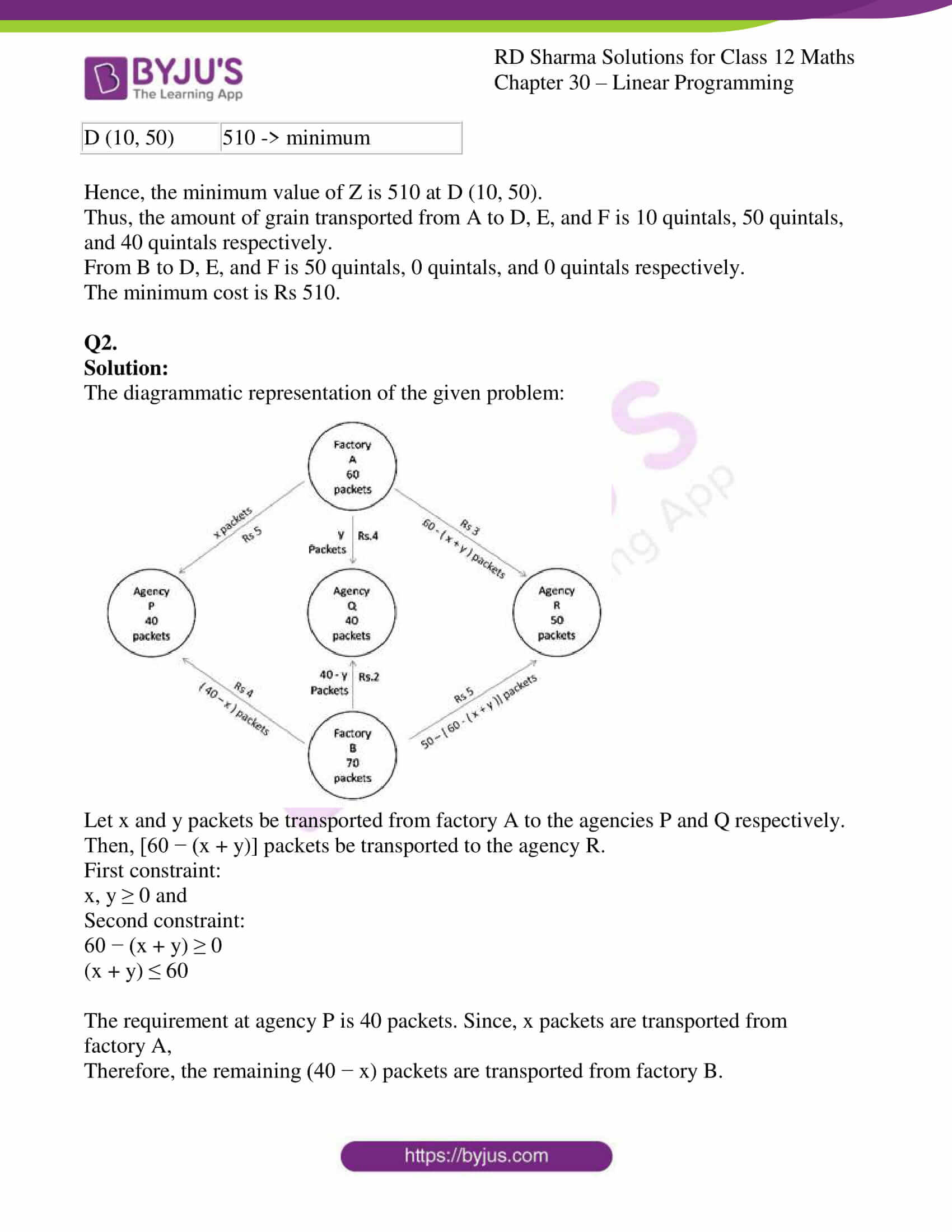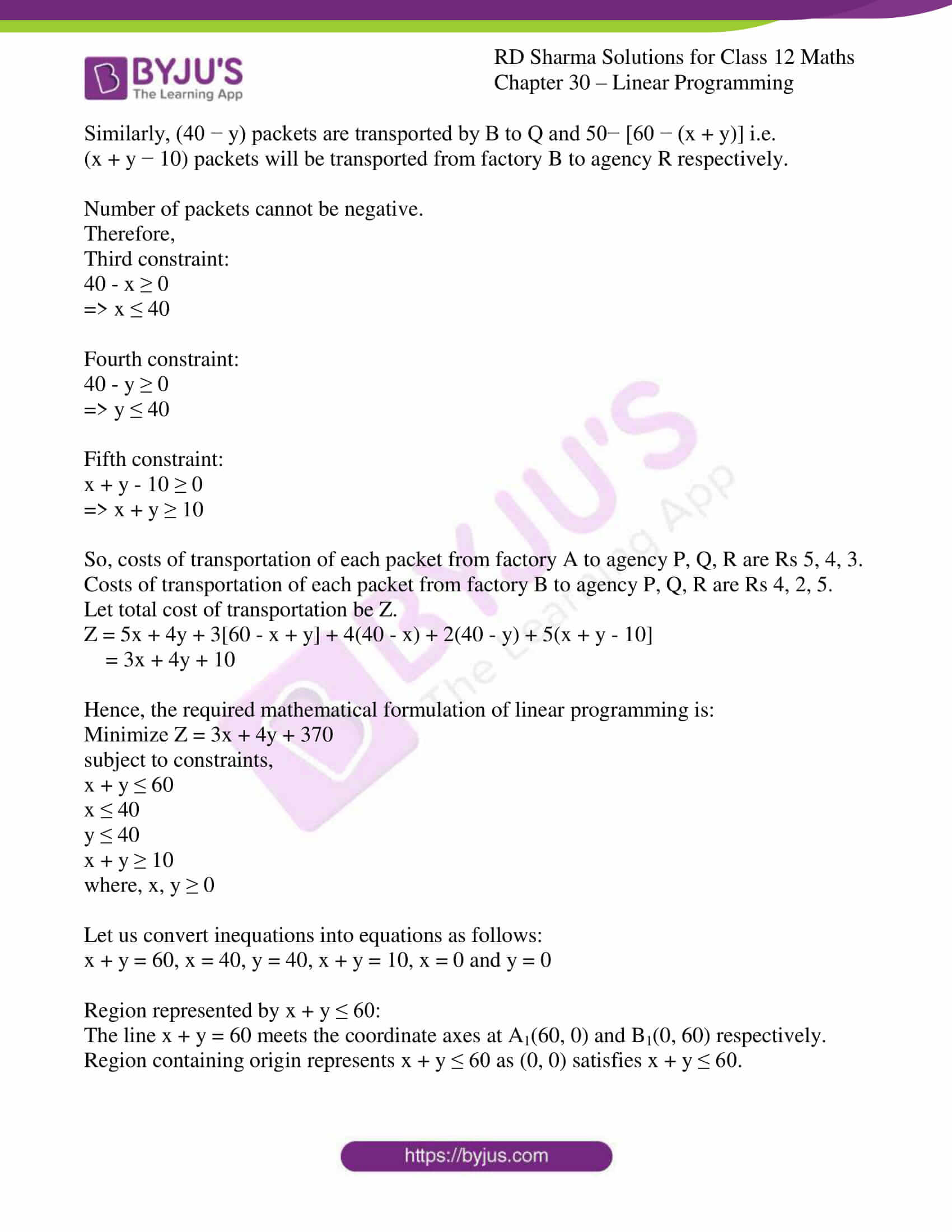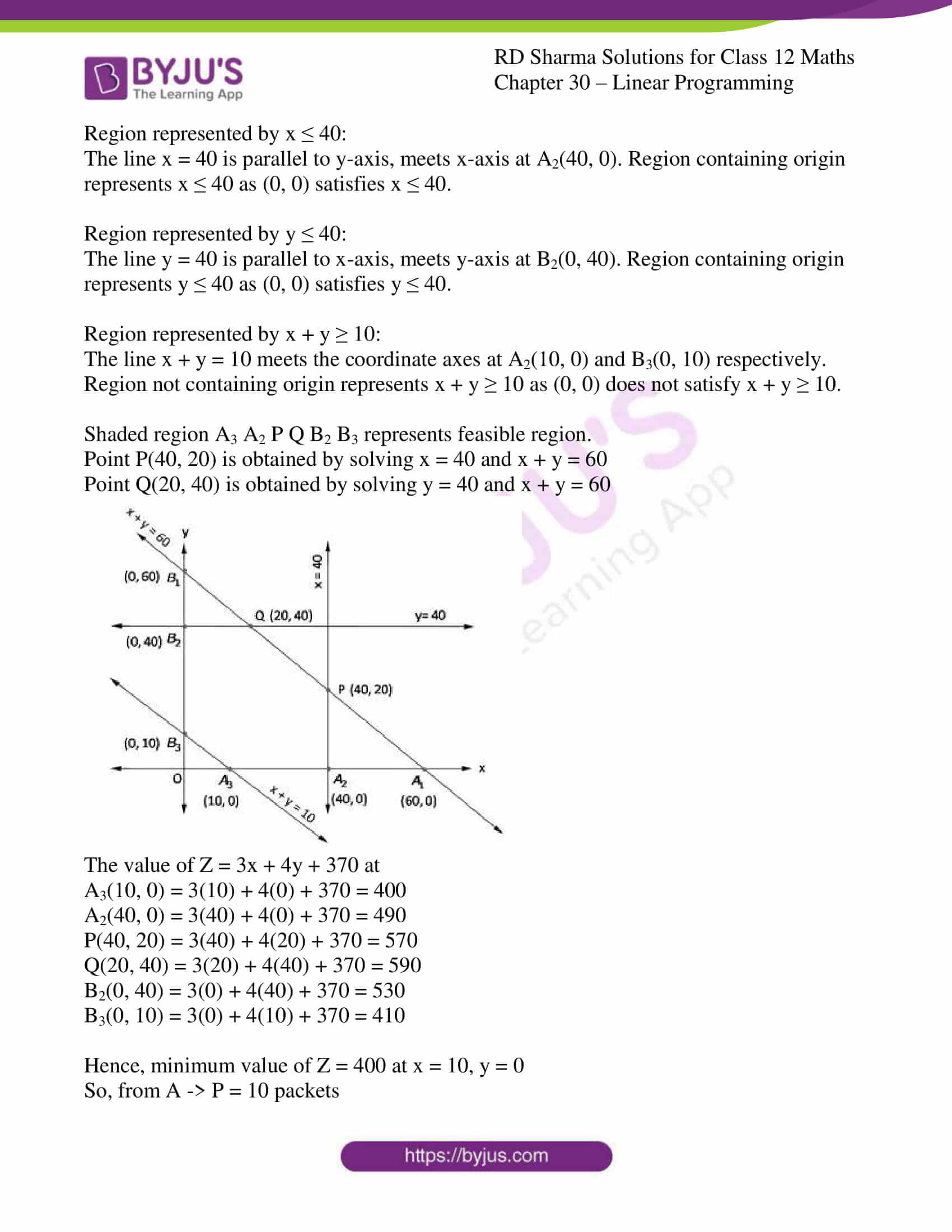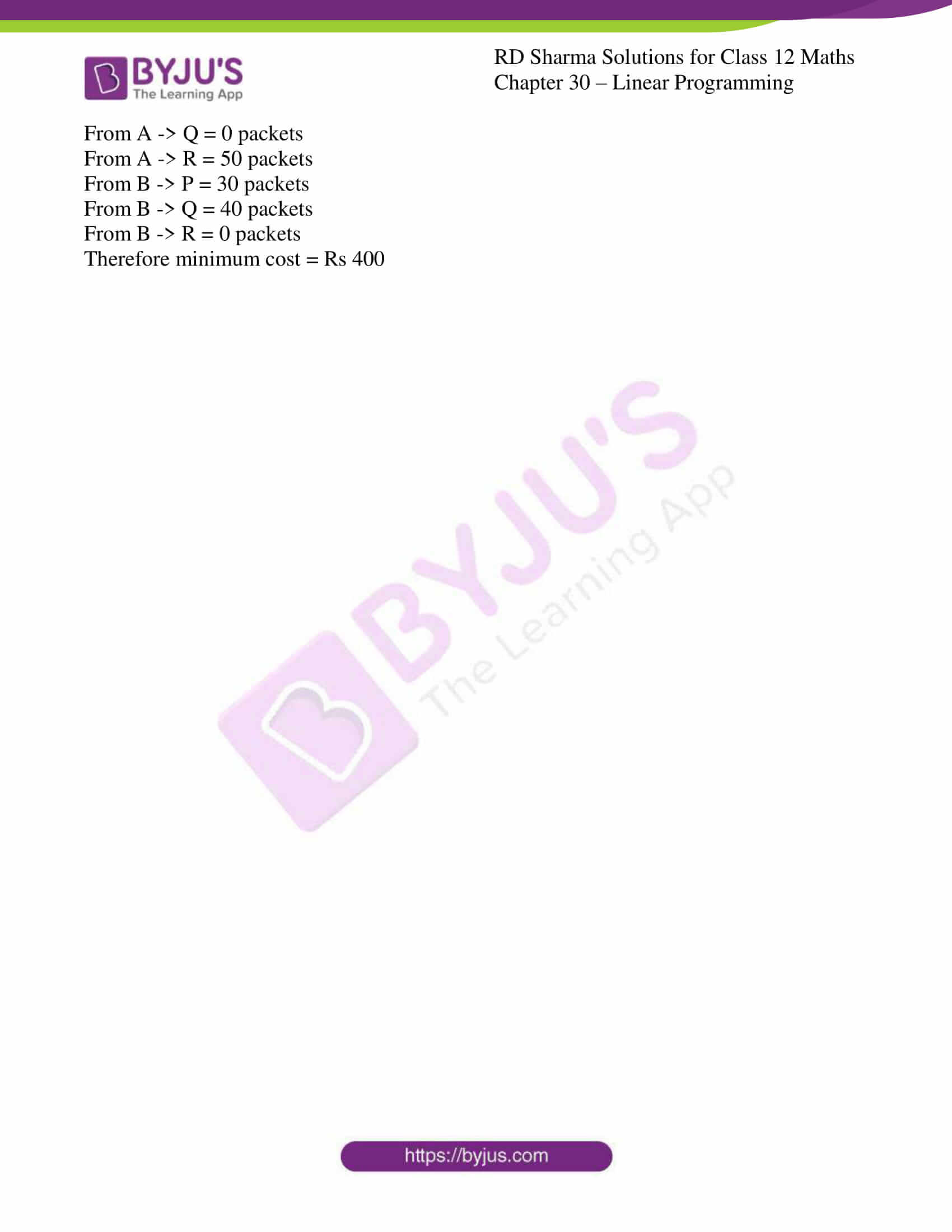### Access RD Sharma Solutions for Class 12 Maths Chapter 30 Exercise 5

EXERCISE 30.5

Q1.

Solution:

Let us consider godown A supply x and y quintals of grain to the shops D and E.

Then, (100 – x – y) will be supplied to shop F.

The requirement at shop D is 60 quintals since, x quintals are transported from godown A.

Therefore, the remaining (60 − x) quintals will be transported from godown B.

Similarly, (50 − y) quintals and 40 − (100 − x − y) i.e. (x + y − 60) quintals will be transported from godown B to shop E and F respectively.

Here is the diagrammatic representation of the given problem: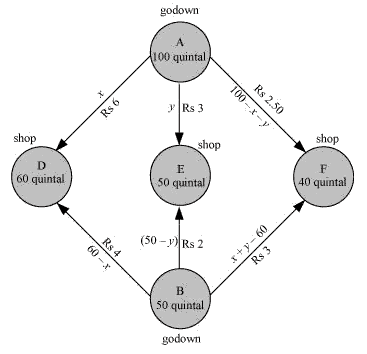x ≥ 0 , y ≥ 0 and 100 – x – y ≥ 0

⇒ x ≥ 0 , y ≥ 0 , and x + y ≤ 100

60 – x ≥ 0 , 50 – y ≥ 0 , and x + y – 60 ≥ 0

⇒ x ≤ 60 , y ≤ 50 , and x + y ≥ 60

Total transportation cost Z is given by,

Z = 6x + 3y + 2.5(100 – x – y) + 4(60 – x) + 2(50 – y) + 3( x + y – 60)

= 6x + 3y + 250 – 2.5x – 2.5y + 240 – 4x + 100 – 2y + 3x + 3y – 180

= 2.5x + 1.5y + 410

Hence, the required mathematical formulation of linear programming is:

Minimize Z = 2.5x + 1.5y + 410

subject to the constraints,

x + y ≤ 100

x ≤ 60

y ≤ 50

x + y ≥ 60

x, y ≥ 0

The feasible region obtained by the system of constraints is: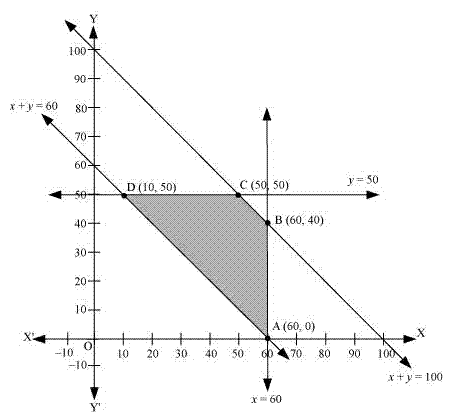The corner points are A(60, 0), B(60, 40), C(50, 50), and D(10, 50).

The values of Z at these corner points are as follows.

 Corner point Z = 2.5x + 1.5y + 410 A (60, 0) 560 B (60, 40) 620 C (50, 50) 610 D (10, 50) 510 -> minimum

Hence, the minimum value of Z is 510 at D (10, 50).

Thus, the amount of grain transported from A to D, E, and F is 10 quintals, 50 quintals, and 40 quintals respectively.

From B to D, E, and F is 50 quintals, 0 quintals, and 0 quintals respectively.

The minimum cost is Rs 510.

Q2.

Solution:

The diagrammatic representation of the given problem: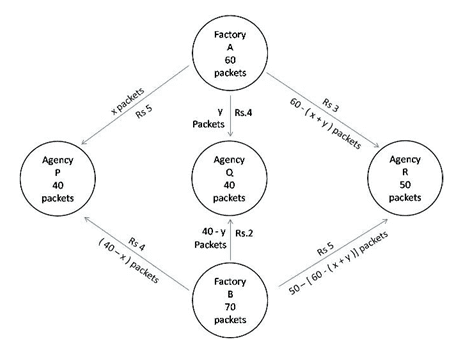Let x and y packets be transported from factory A to the agencies P and Q respectively.

Then, [60 − (x + y)] packets be transported to the agency R.

First constraint:

x, y ≥ 0 and

Second constraint:

60 − (x + y) ≥ 0

(x + y) ≤ 60

The requirement at agency P is 40 packets. Since, x packets are transported from factory A,

Therefore, the remaining (40 − x) packets are transported from factory B.

Similarly, (40 − y) packets are transported by B to Q and 50− [60 − (x + y)] i.e. (x + y − 10) packets will be transported from factory B to agency R respectively.

Number of packets cannot be negative.

Therefore,

Third constraint:

40 – x ≥ 0

=> x ≤ 40

Fourth constraint:

40 – y ≥ 0

=> y ≤ 40

Fifth constraint:

x + y – 10 ≥ 0

=> x + y ≥ 10

So, costs of transportation of each packet from factory A to agency P, Q, R are Rs 5, 4, 3.

Costs of transportation of each packet from factory B to agency P, Q, R are Rs 4, 2, 5.

Let total cost of transportation be Z.

Z = 5x + 4y + 3[60 – x + y] + 4(40 – x) + 2(40 – y) + 5(x + y – 10]
= 3x + 4y + 10

Hence, the required mathematical formulation of linear programming is:

Minimize Z = 3x + 4y + 370

subject to constraints,

x + y ≤ 60

x ≤ 40

y ≤ 40

x + y ≥ 10

where, x, y ≥ 0

Let us convert inequations into equations as follows:

x + y = 60, x = 40, y = 40, x + y = 10, x = 0 and y = 0

Region represented by x + y ≤ 60:

The line x + y = 60 meets the coordinate axes at A1(60, 0) and B1(0, 60) respectively. Region containing origin represents x + y ≤ 60 as (0, 0) satisfies x + y ≤ 60.

Region represented by x ≤ 40:

The line x = 40 is parallel to y-axis, meets x-axis at A2(40, 0). Region containing origin represents x ≤ 40 as (0, 0) satisfies x ≤ 40.

Region represented by y ≤ 40:

The line y = 40 is parallel to x-axis, meets y-axis at B2(0, 40). Region containing origin represents y ≤ 40 as (0, 0) satisfies y ≤ 40.

Region represented by x + y ≥ 10:

The line x + y = 10 meets the coordinate axes at A2(10, 0) and B3(0, 10) respectively. Region not containing origin represents x + y ≥ 10 as (0, 0) does not satisfy x + y ≥ 10.

Shaded region A3 A2 P Q B2 B3 represents feasible region.

Point P(40, 20) is obtained by solving x = 40 and x + y = 60

Point Q(20, 40) is obtained by solving y = 40 and x + y = 60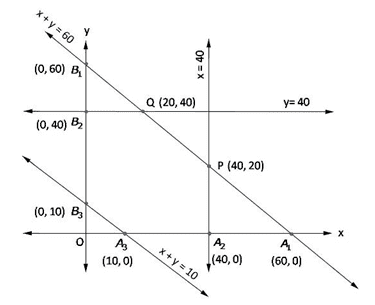The value of Z = 3x + 4y + 370 at

A3(10, 0) = 3(10) + 4(0) + 370 = 400

A2(40, 0) = 3(40) + 4(0) + 370 = 490

P(40, 20) = 3(40) + 4(20) + 370 = 570

Q(20, 40) = 3(20) + 4(40) + 370 = 590

B2(0, 40) = 3(0) + 4(40) + 370 = 530

B3(0, 10) = 3(0) + 4(10) + 370 = 410

Hence, minimum value of Z = 400 at x = 10, y = 0

So, from A -> P = 10 packets

From A -> Q = 0 packets

From A -> R = 50 packets

From B -> P = 30 packets

From B -> Q = 40 packets

From B -> R = 0 packets

Therefore minimum cost = Rs 400